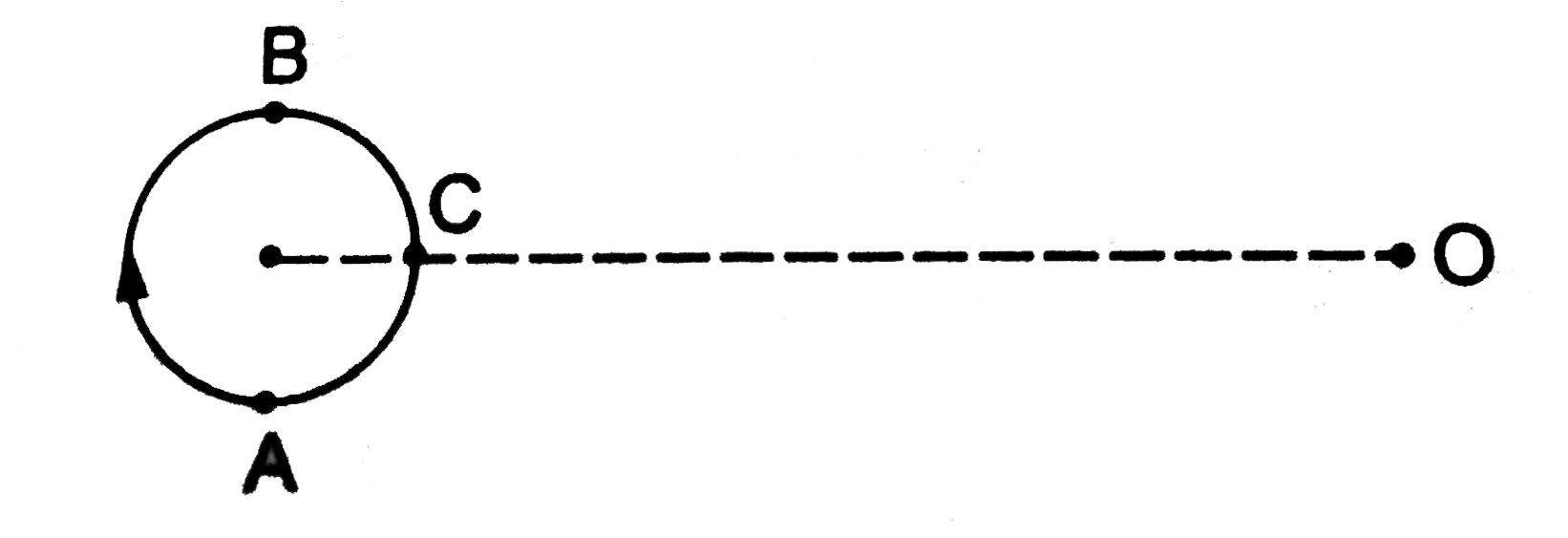# A small source of sound moves on a circle as shown in figure and an observer is sitting at O. Let v_1,v_2,v_3 be the frequencies heard when the sour

30 views
in Physics
A small source of sound moves on a circle as shown in figure and an observer is sitting at O. Let v_1,v_2,v_3 be the frequencies heard when the source is at A,B and C respectively..
A. n_(1)gtn_(2)gtn_(3)
B. n_(2)gtn_(3)gtn_(1)
C. n_(1)=n_(2)gtn_(3)
D. n_(2)gtn_(1)gtn_(3)

by (24.7k points)